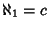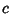## Aleph-1

The Set Theory symbol for the smallest Infinite Set larger than Aleph-0. The Continuum Hypothesis asserts that, whereis the Cardinality of the ``large'' Infinite Set of Real Numbers (called the Continuum in Set Theory). However, the truth of the Continuum Hypothesis depends on the version of Set Theory you are using and so is Undecidable.

Curiously enough,-D Space has the same number of points () as 1-D Space, or any Finite Interval of 1-D Space (a Line Segment), as was first recognized by Georg Cantor.See also Aleph-0, Continuum, Continuum Hypothesis, Countably Infinite Set, Finite, Infinite, Transfinite Number, Uncountably Infinite Set